# What Is An Extraneous Solution? (3 Key Concepts To Know)

When we use algebra to solve equations, we can usually trust all of the answers we get.  However, there are some cases where this is not true – that is, when we get extraneous solutions.

So, what is an extraneous solution?  An extraneous solution for an equation is a value we find when solving the equation that does not satisfy the equation. For example, if we square both sides of the equation √x = -1, we get a result of x = 1, which does not satisfy the original equation, which means x = 1 is an extraneous solution.

Of course, squaring both sides of an equation is not the only way that extraneous solutions can appear.  In fact, some equations can have more than one extraneous solution, depending on how we solve them.

In this article, we’ll talk about extraneous solutions, what they are, and how they appear.  We will also look at several examples to make the concept clear.

Let’s get started.

## What Is An Extraneous Solution?

An extraneous solution is a “false” solution to an equation.  An extraneous solution does not satisfy the original equation, even though we took seemingly valid steps while solving the equation.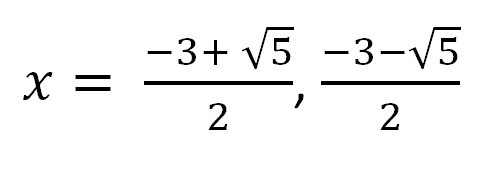Sometimes we find extraneous solutions as a result of steps we took in solving an equation. These values do not satisfy the original equation, which we can see by testing.

### How Do You Know If A Solution Is Extraneous?

You can always tell that a solution is extraneous if it does not satisfy the equation.  That is, take the value of your variable and substitute it back into the original equation.

#### Example 1: Testing For An Extraneous Solution

Recall the example from earlier, when we wanted to solve the equation √x = -1.  After squaring both sides, we get a value of x = 1.

When we substitute x = 1 into the original equation, we get:

• √x = -1  [original equation]
• √1 = -1  [substitute x = 1]
• 1 = -1  [this is not true]

Since the resulting equation is not true, the solution x = 1 must be extraneous. We can verify this by studying the graph below.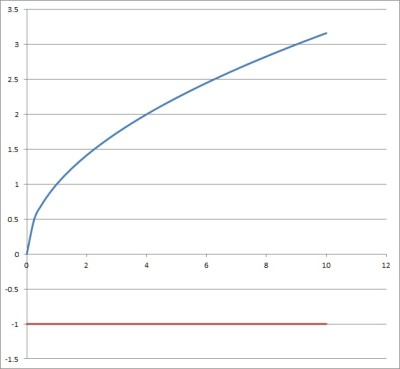This graph compares the radical function y = √x (blue curve) and the horizontal line y = -1 (red line), which never intersect.

*Note: it is true that x = -1 is also a square root of 1.  However, when we see the notation “√1”, we generally take the principal square root of 1, which is 1 (positive 1).

If we mean -1 (negative 1), we write “-√1” to indicate that we want the negative square root.

More generally, write “√x” if you want the positive (principal) square root of x, and “-√x” if you want the negative square root of x.

### Can You Have Two Extraneous Solutions?

It is possible to have two extraneous solutions to an equation.  In fact, you can have three or more extraneous solutions, depending on the equation.

#### Example: An Equation With Two Extraneous Solutions

Consider the equation √(x2 – 9) = -4.  When we solve, we get:

• √(x2 – 9) = -4  [original equation]
• (√(x2 – 9))2 = (-4)2  [square both sides]
• x2 – 9 = 16  [simplify both sides]
• x2 = 25  [add 9 to both sides]
• x = +5, -5

Note: we need to take both the positive and negative square roots to find these two values.

However, both x = 5 and x = -5 are extraneous solutions for this equation.  We can see this by substituting x = -5 back into the original equation:

• √(x2 – 9) = -4  [original equation]
• √((5)2 – 9) = -4  [substitute  x= 5]
• √(25 – 9) = -4
• √(16) = -4
• 4 = -4

This last equation is not true, meaning that x = 5 is an extraneous solution.

The calculations are similar when you plug in x = -5 to find out that is it also an extraneous solution.

So, the equation √(x2 – 9) = -4 has two extraneous solutions. We can verify this by studying the graph below.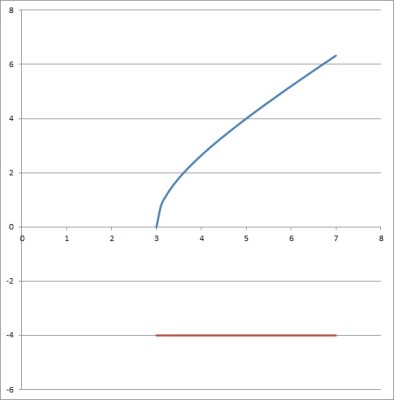This graph compares the radical function y = √(x2 – 9) (blue curve) and the horizontal line y = -4 (red line), which never intersect.

### What Causes An Extraneous Solution?

There are several operations that can cause an extraneous solution to emerge.  For example:

• Radicals – for example, when we square both sides of a radical equation (to cancel a square root), we may introduce extraneous solutions when the radical is equal to a negative number (since there is no solution to that equation (same for a fourth root, sixth root, or any even root).
• Multiplication – for example, when we multiply both sides of an equation by x, we may find the extraneous solution x = 0.  Another example is multiplying both sides of an equation by 0, which makes the equation true in all cases (for any and every value of a variable), thus introducing numerous extraneous solutions.
• Rational Expressions – for example, when working with common denominators to solve equations with rational expressions.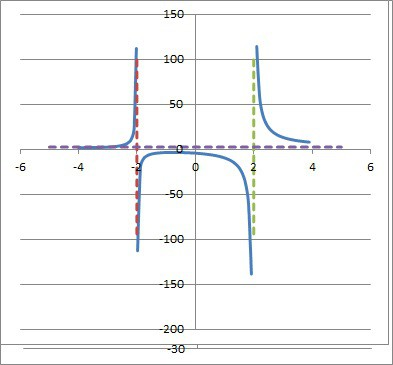Solving rational equations can introduce extraneous solutions (the graph of a rational function has at least one vertical asymptote).

### How Do You Find Extraneous Solutions?

Generally, the best way to find extraneous solutions is to solve the equation and find values for the variables.  Then, check your answers by substituting the values back into the original equation.

#### Example 1: A Radical Equation With Extraneous Solutions

Consider the equation √(x2 – 36) = -8.  When we solve, we get:

• √(x2 – 36) = -8  [original equation]
• (√(x2 – 36))2 = (-8)2  [square both sides]
• x2 – 36 = 64  [simplify both sides]
• x2 = 100  [add 36 to both sides]
• x = +10, -10

Note: we need to take both the positive and negative square roots to find these two values.

However, both x = 10 and x = -10 are extraneous solutions for this equation.  We can see this by substituting x = -5 back into the original equation:

• √(x2 – 36) = -8  [original equation]
• √((10)2 – 36) = -8  [substitute  x = 10]
• √(100 – 36) = -8
• √(64) = -8
• 8 = -8

This last equation is not true, meaning that x = 10 is an extraneous solution.

The calculations are similar when you plug in x = -10 to find out that is it also an extraneous solution.

So, the equation √(x2 – 36) = -8 has two extraneous solutions.

#### Example 2: Extraneous Solutions When Multiplying By Zero

Consider the equation x = 2.  It has only one solution.

However, if we multiply by zero on both sides, we get:

• 0*x = 0*2
• 0 = 0

This equation is true in all cases, regardless of the value of x.  In multiplying by zero on both sides of the equation, we have introduced every real number (except x = 2) as an extraneous solution.

#### Example 3: Extraneous Solutions When Multiplying By x

Consider the equation x – 5 = 0.  It has only one solution: x = 5.

However, if we multiply by x on both sides, we get:

• x*x – x*5 = x*0
• x2 – 5x = 0
• x(x – 5) = 0
• x = 0 or x = 5

However, when we substitute x = 0 into the original equation, we get:

• x – 5 = 0  [original equation]
• 0 – 5 = 0  [substitute x = 0]
• -5 = 0

This last equation is not true.  In multiplying by x on both sides of the equation, we have introduced x = 0 an extraneous solution.

In general, if we multiply an equation by x – r, we may introduce x = r as an extraneous solution.

#### Example 4: A Rational Equation With An Extraneous Solution

Consider the following rational equation:

• 1/(x-1) + 2/(x+1) = 2/(x2 – 1)

We can factor x2 – 1 as a difference of squares to get (x+1)(x-1).  So, we can cancel all denominators in this equation if we multiply both sides by x2 – 1.

This leaves us with the following equation to solve:

• 1(x+1) + 2(x-1) = 2
• x + 1 + 2x – 2 = 2
• 3x – 1 = 2
• 3x = 3
• x = 1

However, if we substitute x = 1 back into the original equation, we get zero denominators in the first (leftmost) term and the rightmost term:

• 1/(x-1) + 2/(x+1) = 2/(x2 – 1)  [original equation]
• 1/(1-1) + 2/(1+1) = 2/(12 – 1)  [substitute x = 1]
• 1/(0) + 2/(2) = 2/(0)

Since we cannot resolve the equation (due to zero denominators), we conclude that x = 1 is an extraneous solution for this equation.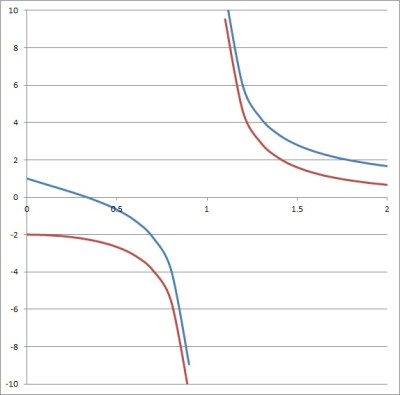We can see from this graph that x = 1 is an extraneous solution to the original equation mentioned above in this example.

### Why Is It Important To Check For Extraneous Solutions?

It is important to check for extraneous solutions because they do not really satisfy the equation.  These values only emerge as a result of a step we took when solving the equation.

It is a good idea to check your answers by substituting values back into the original equation.  This allows you to detect extraneous solutions and also ensures that you did not make a calculation mistake somewhere along the way.

## Conclusion

Now you know what extraneous solutions are and how they can appear when solving an equation.  If you stay mindful of these possibilities when solving equations, you should be able to prevent or detect extraneous solutions.